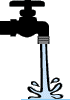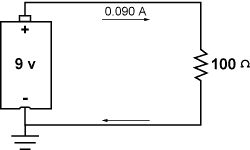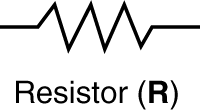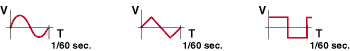# Attributes of Electricity

### Voltage and current

Electricity has two primary attributes: voltage and current. Voltage and current are separate properties, but are interrelated in electronic circuits. Both must be present for proper circuit operation.

Voltage is the force that causes current to flow in a circuit. It is measured in volts (V or E). To get a better sense of voltage, think about a water faucet. Voltage can be compared to the pressure at which the water comes out of the faucet. Consider a faucet from which water flows in a slow, steady stream. This would be analogous to a low voltage circuit. A fire hose, which sprays water with a great force, can be considered analogous to a high voltage circuit. In all electronic circuits, there must be voltage in order to have current flow.Faucet Fire Hose

Now take a look at current in electronics. Current is the movement of electrical charges, the flow of electrons through the electronic circuit. Current is measured in amperes or amps (A or I). Go back to the faucet; think of current as the amount of water coming out. The more water going through the faucet in an hour, the higher the current. Think of it as like a river. After a rain, the river starts flowing much harder. It is said to have a stronger current because there is more water passing a given point in a set amount of time. Current and voltage go hand in hand. The higher the voltage, the higher the current. One thing that can change this is how much resistance is in the circuit. Electronic components are designed to handle a certain amount of voltage and current. These are factors determined by the manufacturer.

Now consider a D-cell battery. It has a positive terminal (the top side with the button sticking out) and a negative terminal (the bottom side with the button going in). If a wire is connected from one pole to the other, there will be current flowing through the wire. Current flows as a result of what is called an electromagnetic potential (the voltage), or a potential difference between two points. The positive side of the battery is considered to have a potential of 1.5 volts, while the negative side of the battery is considered to have a potential of zero volts. There is a potential difference of 1.5 volts between the terminals of the battery. Current will flow in the wire from the positive terminal to the negative, or from the positive potential to the zero potential. Ground, a commonly used term in electronics, is always considered to be at zero volts, and the term ground is often used instead of zero volts. In the example above, the negative terminal of the battery is the ground, at zero volts, though negative and ground are not always the same thing.

Again go back to the water faucet to get a better understanding of this. Imagine there are two faucets with hoses connected to them. They have the same water pressure coming out of both faucets. Connect the two hoses together and turn on the faucets. What does the water do? If there is the same amount of pressure through the faucets, there is no potential difference; no one force is stronger than the other, so there is no flow. Remove pressure from one faucet, and water will flow through the hose from the faucet with pressure to the faucet with no pressure, just as electric current flows from the positive terminal of the battery to the negative terminal of the battery.Battery and resistor circuit

### Resistance

Resistance is a phenomenon of nature, just as voltage and current are. Just as voltage is the force that causes electrons to flow, and current is the quantity of electron flow, resistance is the resistance to electron flow. Again the faucet and hose analogy applies well. Consider a faucet with a garden hose attached to it. The garden hose has a certain area through which water can flow; this can be considered the resistance of the hose. If the hose is bent, the flow of the water will lessen, even to the point of stopping. Bending the hose makes the diameter smaller; this increases the resistance to the flow of water. In electronic circuits, resistance is supplied by devices called resistors, and the value of resistors is measured in ohms (W).Symbol for resistor

Resistors provide the final balancing element in the basic electric circuit, which must have three elements to operate: voltage, current, and resistance. The resistor provides the load, or the basic element upon which work is done. In a practical circuit, a load might consist of an electric motor, or a light bulb, or a pair of speakers. All of these loads have a certain resistance associated with them.

Consider the simple circuit above. It consists of a 9-volt battery, a 100-ohm resistor, and a wire through which flows a 0.090 amp current. These numbers are arrived at through Ohm's law, which reads V = IR, where V is voltage, I is current, and R is resistance. Ohm's law is a simple algebraic equation. One can calculate any one of the three variables, V, I, or R, if given the other two. In most electronic situations, the voltage is given, and one must calculate either the resistance or the current. In the example above, consider the voltage and resistance as given.

V = 9.0
R = 100
V= IR

Dividing both sides of the equation by R results in
I = V/R = 0.09 amps

### Electric power

Power is a measure of how much energy is being used at any given instant, or how much work is being done at any given time. In electronics, power is expressed in terms of watts, which result from the voltage and the current. In short, power (P) is equal to voltage times current, P = VI. Power is important in electronic circuits in many respects. A circuit must have enough power to drive a given load. Just as a big truck has enough power to pull a big boat, and a VW bug does not, a circuit with the appropriate power output must be chosen for the correct load. Power considerations are also important when specifying electrical connections. One must often make sure a building's electrical system has enough power supplied to power all the devices connected to it. To calculate the power of the system of the previous example, use the equation given here, P = VI.

P = VI = 9.0V x 0.09A = 0.810 watts

### AC and DC electricity and waveforms

Electronic circuits operate on a number of different types of voltage and current, typically categorized as AC or DC. The example used above of a battery working with a resistor is an example of a DC circuit. DC stands for "direct current", and refers to a current or a voltage level that does not change with time. In the example of the 9-volt battery, the battery is always at 9 volts. The voltage does not change as time passes, so neither will the current.

Many voltage or current sources are called AC. AC stands for "alternating current", and refers to current and voltage that vary with time. The most common source for AC voltage and current is the electrical outlet on your wall. It supplies a sinusoidal voltage source that moves smoothly from -110 to +110 volts, 60 times every second. There are many other AC wave forms, the square wave and triangular wave among them, but the sine wave is the most common.Sine, Triangle, and Square Waves (respectively)

Both AC and DC voltages are necessary in electronics. Signals that carry information, such as video or audio signals, are by their very nature AC. The information they carry is represented by changes in amplitude, frequency, and phase of the waveform. DC voltage and current sources are typically used to power active electronic components such as amplifiers or processors.